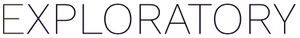# How can I get "Yes" if the target column value is in a certain range, otherwise "No"?

#1

Suppose you have a “Value” column with number data, and if you want to add a new column “IsInRange” that contains “Yes” if the corresponding value is between 10 and 16, otherwise “No” like following.

Here is how to do it. First, you can select “Mutate” menu from the column header menu.

then, set the column name and type in like following in the Calculation Editor.

``````ifelse (between(Value, 10, 16), "Yes", "No")
``````

That’s it!

The `between` is a function that returns TRUE if the value is in a given range. Otherwise, it returns FALSE. The 1st argument is a column name (Value). 2nd and 3rd arguments are the min and max of the range. Note that it is inclusive. For example, if you set 10 and 16 for the range, it returns TRUE for the value “10” and “16”.

The `ifelse` function takes 3 arguments. If the 1st argument returns TRUE, it returns the 2nd argument (“Yes” in this example), Otherwise it returns the 3rd argument (“No” in this example).

Note that you can use the `between` function not only for number data, but for date data. Here is an example of getting “Yes” if the value in `start_date` column is in between 1/3/2011 and 1/10/2011.

``````ifelse(between(start_date, as.Date("2011-01-03"), as.Date("2011-01-10")), "Yes", "No")
``````